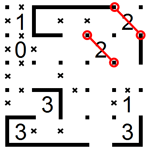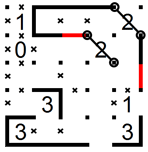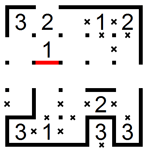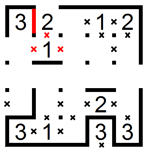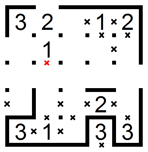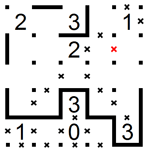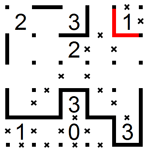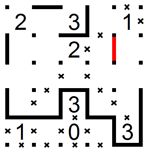ВходРегистрация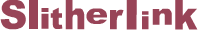Slitherlink is a loop-forming puzzle based on a rectangular lattice of dots containing clues in various places. The object is to link adjacent dots so the value of each clue equals the number of links surrounding it and the solution forms a single continuous loop with no crossings or branches when the puzzle is completed. In this example we have a 5x5 Slitherlink puzzle with five columns and five rows. We now need to create a continuous loop according to the above rules… but how?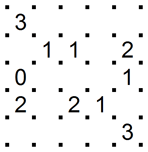## Starting techniques

Slitherlink puzzles always start off with some very easy moves, many of which are obvious at first glance. After solving the first clues, proceed to basic techniques to get more ideas how to solve the puzzles. Here are some ways of using the starting techniques:

#### 1. No lines around a 0:

When solving Slitherlink puzzles it is equally important to eliminate links where lines are NOT allowed. The best way to do this is by marking them with an X. Let’s look at the 0 in the center of this example. The 0 means it is not surrounded with any lines so we can place four X’s around it to show all four links are excluded. This example also shows a 0 in a corner and a 0 on a side. In both cases, two additional X’s are marked to show that lines are not allowed because they cannot be continued.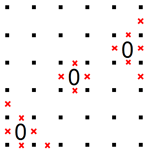#### 2. Adjacent 0 and 3:

In this example there is an X above the 3, so there is only one way to loop around the 3: on the left, on the bottom and on the right as shown below. We can now extend the loop with two additional lines, one to the right and one to the left, because there is only one way to continue in each direction. And finally, we can mark four X’s next to the corners because having lines in these places will create branches (or crossings), which are not allowed according to Slitherlink rules.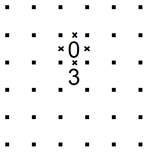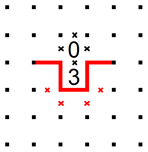#### 3. Diagonal 0 and 3:

The combination of 0 and 3 also creates special starting technique situation when located diagonal to each other. In this example the gray lines show the only two possible solutions. Any other way would lead to a conflict later on. Since two lines are common to both possibilities we know they must be part of the loop in the puzzle solution.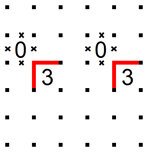There are only two solutions for adjacent 3’s, as shown with the gray lines in this example. Any other way of drawing the lines will quickly lead to a conflict. Since three of the red lines are common to both solutions we know they must be part of the loop. We also see that, regardless of which solution we end up with, the loop must always bend between the 3’s. This means that two X’s can be marked as well. This technique works the same way for any number of adjacent 3’s.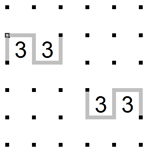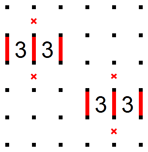#### 5. Two diagonal 3's:

There are several ways to loop around two diagonal 3’s, one of which is shown with the gray lines below. However, there are four lines shown in red common to all solutions and therefore must be part of the loop. To avoid crossing and branching, four X’s are also marked. Excluding these links will be essential later on for the progress of the puzzle.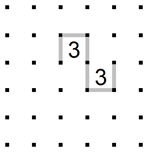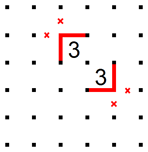#### 6. Any number in a corner:

Any number in a corner will always provide some immediate starting points. The 0 is simple and has been described in starting technique 1. The 1 requires placing two X’s in the corner because it is not possible to place just one line in that area. The 2 has two solutions both of which connect the dots circled in red so the two red lines must be part of the loop. And finally, there are only two ways to loop around the 3 and the red lines show which lines are common to both solutions.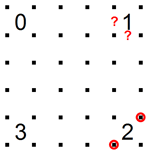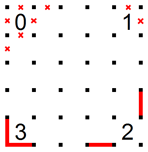## Basic techniques

The next step in Slitherlink is to continue the partially solved lines. In some cases the progress is quick and easy because there is only one alternative while in other cases there are several alternatives making further analysis necessary. Here are some ways of using the basic techniques:

#### 1. Constraints on a 3:

Because 3 is the largest clue in Slitherlink requiring lines around three of its sides, it often happens that a neighboring constraint helps see what some of these lines should be. In this example we have a 3 on the bottom edge of the puzzle, constrained on the right by an X. Similar to the corner situation of starting techniques, the red lines show the paths common to all possible solutions, and which therefore must be part of the loop.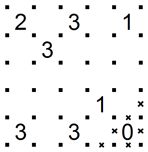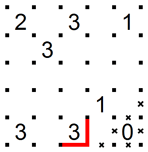#### 2. Loop reaching a 3:

In this example the top part of the loop reaches the corner of a 3 with the possibility to continue in three directions: to the left, down, and to the right. However, the loop can continue around the 3 in only two possible ways. The red lines show paths common to the two possible solutions, and which therefore must be part of the loop. Since the loop must continue around the 3, it cannot branch to the left and we must therefore add X to show that this link is excluded.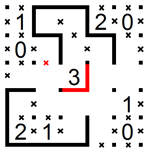#### 3. Loop reaching a 1:

Sometimes the loop reaches a 1 in such a way that it is forced to continue on one of its sides. In this example we see the loop reaching 1 on the bottom edge of the puzzle. As a result, the loop can continue either upwards or to the right. This means the links on the right and on the top of the 1 are excluded, as shown with the red X’s.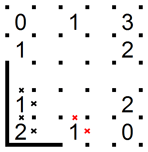#### 4. Constraints on a 2:

Some of the most interesting situations in Slitherlink occur when the loop goes around a 2. By carefully examining this example one can see there are only two ways the loop can go around the 2. However, both solutions will always connect the two dots circled in red. This means there is only one way the loop can continue as shown with the red line.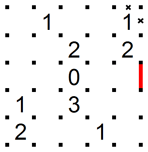#### 5. Avoiding a separate loop:

The rule of Slitherlink says only one loop may be formed. This means we must never close smaller loops in the puzzle. In this example we have three ways to continue the loop in the bottom part of the puzzle: to the left, upwards and to the right. However, if we make a line to the left a separate loop will be formed which is not allowed. Therefore this link is excluded with an X.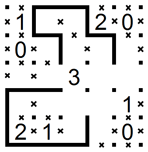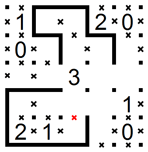The techniques described so far won’t be enough to solve hard puzzles. For this you will need advanced techniques to work out many special and interesting logic situations. Most advanced techniques use recursion, a looking ahead process of making assumptions and checking for conflicts one or two steps ahead. Here are some examples of advanced techniques to solve special situations. You will develop many more of your own when solving hard Slitherlink puzzles by yourself:

If the loop in the bottom-left corner is continued upwards, as shown in the left diagram, the next steps will quickly create a closed loop conflict. Therefore the loop cannot be continued upwards as marked with the X.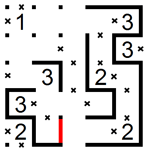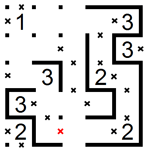If we mark an X next to the 3 in the left diagram we will then have to add three lines around it as shown in the center diagram which creates conflict with the 3 in the top-right corner. Therefore there should be a line instead of the X as shown in the right diagram.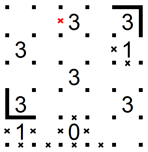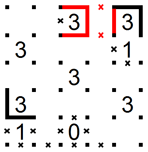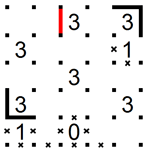If the loop in the bottom-right corner is continued upwards as shown in the left diagram, we will be forced to create a small loop as shown in the center diagram. Therefore the loop cannot continue upwards and an X should be marked X instead.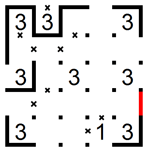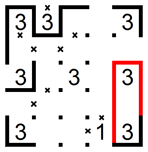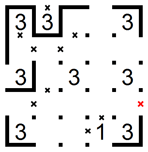The two red links in the left diagram show how the loop goes around the 2’s in the top-right corner. We don’t know if the loop goes inwards to the center or outwards to the corner of the puzzle, but we do know the loop must connect to the dots with the small red circles. This means the two red lines shown in the right diagram are part of the solution.